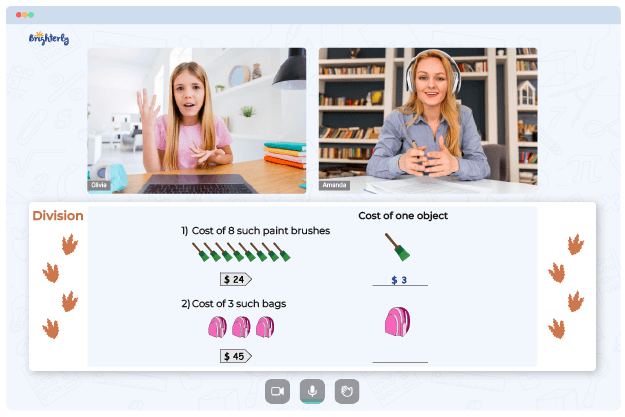# Math Worksheets 2 Digit Divisors

Math can be challenging when students are at a loss or lack the proper math foundation. So, how can you make the process easier for kids?

## Why Brighterly’s Tutors Use Math Worksheets 2 Digit Divisors

Brighterly is an online math platform with tutors willing to help kids love math. The tutors at Brighterly use long division with 2-digit divisor worksheets that allows kids to understand the topic better.

Math for Kids

Is Your Child Struggling With Math?
1:1 Online Math TutoringThe division with 2-digit divisors 5th grade worksheets will help students practice division with the remainder, both in standard form and in the context of word problems. Tutors encourage kids to apply the long division technique to divide 2-digit whole numbers using division with 2 digit divisor worksheets.### Math Worksheets 2 Digit Divisors PDF

2 Digit Divisor Worksheets### Math Worksheets 2 Digit Divisors PDF

Long Division With 2-Digit Divisors Worksheet### Math Worksheets 2 Digit Divisors PDF

Division With 2-Digit Divisors 5Th Grade Worksheets### Math Worksheets 2 Digit Divisors PDF

Division With 2 Digit Divisor Worksheets

In grades three and four, students are encouraged to solve each division issue by dividing, multiplying, subtracting, and bringing down to get the quotient and remainder. Also, a long division with 2-digit divisors worksheet comes in lovely colors that capture kids’ attention.

### More Division Worksheets

Need help with Division?• Does your child need extra support with understanding of division?
• Start lessons with an online tutor.

Is your child finding it hard to grasp division basics? An online tutor could be of help.